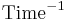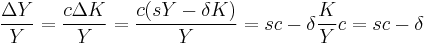# Harrod–Domar model

The Harrod–Domar model is a long-run economic growth model.

## Variables in the model

Name Variable Unit Rival input? Variable type Notes
Output Y Units of GDP (dollar?) Endogenous
Physical capital (capital stock) K Yes Endogenous Physical capital includes things like machines, computers, buildings, etc.
Consumption C
Investment I
Amount saved S
Growth of X$g_X = \dot{X}/X = \frac{\frac{\partial X}{\partial t}}{X}$$\text{Time}^{-1}$
Depreciation (rate?) δ, d, D Unitless
Capital per worker k = K/L Endogenous
Fraction saved s Unitless
Time t Time, e.g. years
Production function F

## Mathematical formalism

### Discrete$\frac{\Delta Y}Y = \frac{c \Delta K}Y = \frac{c (sY - \delta K)} Y = sc - \delta \frac KYc = sc - \delta$

### Continuous$\dot Y / Y = \dot K / K = (sY - \delta K) / K = sY/K - \delta = sc - \delta$

## Commentary

Easterly in The Elusive Quest for Growth criticizes this model.